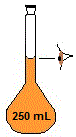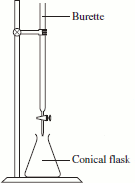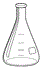Redox Titration of Bleach Chemistry Tutorial

Key Concepts

• Commercially available solutions of bleach contain sodium hypochlorite, NaClO(aq).
• Sodium hypochlorite, NaClO, is a very pale yellow liquid.
• Hypochlorite, ClO-(aq), is the bleaching agent in bleach.
It acts by oxidizing the "stain molecules" to less-coloured forms of the molecules.
In the process, chlorine is reduced from an oxidation state of +1 in ClO- to -1 in Cl-
• It is possible to determine the concentration of hypochlorite in bleach in the laboratory using a 2 step process:
1. Hypochlorite is converted to chloride in the presence of excess iodide, producing brown-coloured iodine molecules.
2. Redox titration of iodine molecules using aqueous sodium thiosulfate solution.
• Note that sodium thiosulfate, Na2S2O3(aq), is not generally used as a primary standard, so it should be standardised using a suitable primary standard such as potassium iodate, KIO3(aq).

No ads = no money for us = no free stuff for you!

Theory

A good method for the direct analysis of hypochlorite, ClO-(aq), does not exist.
Therefore, the hypochlorite in the bleach solution is first reacted with an excess of iodide ion under acidic conditions1.

 reduction of chlorine: ClO-(aq) + 2H+(aq) + 2e → Cl-(aq) + H2O(l) oxidation of iodine: 2I-(aq) → 2e + I2(aq) redox reaction: ClO-(aq) + 2H+(aq) + 2I-(aq) → Cl-(aq) + H2O(l) + I2(aq) name hypochlorite acid iodide chloride water iodine colour pale yellow colourless colourless colourless colourless red-brown

Note that the reaction goes to completion so the moles of brown I2 produced in this reaction is equal to the moles of hypochlorite, ClO-, present in the bleach solution.

We can determine the amount in moles of I2 in the solution by titration with standardised sodium thiosulfate, Na2S2O3(aq).

 oxidation of sulfur: 2S2O32-(aq) → S4O62-(aq) + 2e- reduction of iodine: I2(aq) + 2e- → 2I-(aq) redox reaction: I2(aq) + 2S2O32-(aq) → S4O62-(aq) + 2I-(aq) colour brown colourless colourless colourless

With each addition of thiosulfate, the concentration of I2 decreases, and the brown colour will fade to a pale yellow colour then to colourless, making detection of the end point difficult.
So, a small amount of starch solution is added near the end point of the titration, when the solution is pale yellow2.
Iodine forms a deep blue coloured complex with starch that is easy to see.
At the end point of the titration, the solution will change colour from deep blue to colourless.

Note that at the end point of the titration, moles I2 = ½ × moles S2O32-

Calculations

1. Calculate the average titre of S2O32- in litres, V(S2O32-)
2. Calculate moles of S2O32-, n(S2O32-) = c(S2O32-) x V(S2O32-)
3. Calculate moles of I2: n(I2) = ½ x n(S2O32-)
4. Calculate moles of ClO- in bleach sample: n(ClO-) = n(I2)
5. Calculate concentration of ClO- in bleach sample: c(ClO-) = n(ClO-) ÷ V(ClO-)

Do you know this?

Play the game now!

Preparing the Standard Solution of Potassium Iodate (KIO3)

Potassium iodate, KIO3 can be used as a primary standard.

Procedure
1. Place about 2 g of potassium iodate, KIO3(s), on a watchglass in an oven at 120oC for 2 hours.

2. Transfer the dry potassium iodate to a dessicator to cool.

3. Accurately weigh out about 1.75 g of the cool, dry potassium iodate.

4. Transfer this potassium iodate to a clean 250.00 mL volumetric flask and make up to the mark with deionised water.Calculate the concentration of the potassium iodate standard solution in mol L-1.

1. Calculate the moles of potassium iodate in solution:
moles of solute = mass of solute in grams ÷ molar mass of solute in g mol-1

n = m ÷ M

where:
n = moles of solute in mol
m = mass of solute in g
M = molar mass of solute in g mol-1

In our experiment:
m = mass of potassium iodate (KIO3) = 1.750 g
M = molar mass of potassium iodate (KIO3) = 39.10 + 126.9 + (3 x 16.00) = 214.0 g mol-1
n = moles of potassium iodate (KIO3) = m ÷ M = 1.750 ÷ 214.0 = 8.178 × 10-3 mol

2. Calculate the concentration of the aqueous potassium iodate solution in mol L-1:
concentration of solution = moles of solute ÷ volume of solution in litres
c = n ÷ V

where:
c = concentration of solution in mol L-1
n = moles of solute in mol
V = volume of solution in L

In our experiment:
n = moles of KIO3 = 8.178 x 10-3 mol
V = 250.00 mL = 250.00 ÷ 1000 mL/L = 0.2500 L
c(KIO3(aq)) = n ÷ V = 8.178 x 10-3 ÷ 0.2500 = 0.03271 mol L-1

Do you understand this?

Take the test now!

Standardising the Sodium Thiosulfate Solution (Na2S2O3)

Prepare an aqueous solution of sodium thiosulfate with a concentration of about 0.2 mol L-1:

Preparing Na2S2O3(aq)
1. Weigh out approximately 12.5 g of sodium thiosulfate pentahydrate crystals (Na2S2O3.5H2O(s))
2. Transfer the sodium thiosulfate pentahydrate to a clean 250.00 mL volumetric flask.
3. Make the solution up to the mark with deionised water.
Safety:

Thiosulfate is mildly toxic.

Avoid contact.

Standardise the sodium thiosulfate solution prepared above by titration with the potassium iodate primary standard.

Procedure
1. Fill a 50.00 mL burette (buret) with the sodium thiosulfate solution prepared above.
2. Pipette a 25.00 mL aliquot of the standard potassium iodate solution into a 250 mL conical (erlenmeyer) flask.

3. Add 10 mL of 10% sulfuric acid solution and 2 g of potassium iodide (KI) to the flask.
The solution will appear yellowy-orange.
4. Add sodium thiosulfate solution from the burette to the potassium iodate solution in the flask until the colour starts to fade and becomes straw-coloured.
5. Add a little starch to the conical flask. The solution will turn deep blue.
6. Continue adding sodium thiosulfate solution drop-wise to the conical flask while swirling the flask until the solution turns colourless.
7. Repeat the titration until concordant titres are achieved.Safety:
Iodide and thiosulfate are mildly toxic, avoid contact.
Acids are corrosive, avoid contact.

Use the results of your titration to calculate the concentration of the sodium thiosulfate solution.

1. Calculate the average titre of sodium thiosulfate solution:

Sample Results
Trial No. titre / mL comments
1 25.69 rough: disregard
2 24.96
3 24.98
4 24.97

Calculate the average titre using the three concordant titres:

average titre = (24.96 + 24.98 + 24.97) ÷ 3 = 24.97 mL

2. Calculate moles of iodate ion, IO3-(aq), using known concentration of potassium iodate solution, KIO3(aq), and the aliquot volume:

moles IO3-(aq) = moles of KIO3(aq) = concentration KIO3(aq) in mol L-1 x volume in L
n(IO3-) = c(KIO3) x V(KIO3)
where
V(KIO3) = aliquot volume in L = 25.00 mL = 25.00 ÷ 1000 = 0.02500 L
c(KIO3) = 0.03271 mol L-1
n(IO3-) = 0.03271 x 0.02500 = 8.178 x 10-4 mol

3. Calculate moles of thiosulfate ions consumed in reaction:
The balanced chemical equation for this redox reaction is:
IO3-(aq) + 6H+(aq) + 6S2O32-(aq) → I-(aq) + 3S4O62-(aq) + 3H2O(l)

1 mole IO3-(aq) reacts with 6 moles S2O32-(aq)
therefore 8.178 x 10-4 mol IO3-(aq) reacts with 6 x 8.178 x 10-4 = 4.907 x 10-3 mol S2O32-(aq)

4. Calculate concentration of Na2S2O3(aq) using the average titre:

c(Na2S2O3(aq)) = n(Na2S2O3(aq)) ÷ V(Na2S2O3(aq))

where:
n(Na2S2O3(aq)) = 4.907 x 10-3 mol
V = average titre = 24.97 mL = 24.97 ÷ 1000 = 0.02497 L
c(Na2S2O3(aq)) = 4.907 x 10-3 ÷ 0.02497 = 0.1965 mol L-1

Can you apply this?

Join AUS-e-TUTE!

Do the drill now!

Titrating the Bleach

Commercial bleach is about 0.7 mol L-1, or 5.25% by mass, NaClO.

Step 1: Dilute the Bleach Sample

The first step in the determination of hypochlorite in bleach is to dilute the commercial bleach by a factor of 10.

Procedure
1. Pipette a 25.00 mL aliquot of commercial bleach into a clean 250.00 mL volumetric flask.

2. Make the solution up to the mark using deionised water.

3. Clearly label the volumetric flask as 1:10 commercial bleach
Safety:

Hypochlorite solutions make white spots on coloured clothing, and erode eye and skin tissue.
Aviod contact.

Step 2: React Hypochlorite with Iodide to Produce Iodine

The end point for the titration of hypochlorite with thiosulfate is not easy to detect.
Therefore, an excess of acidifed potassium iodide solution is added to the hypochlorite solution.
All the hypochlorite ions, ClO-(aq), are converted to chloride ions.
And colourless iodide ions are oxidised to brown iodine molecules.

Procedure

1. Pipette a 25.00 mL aliquot of diluted bleach sample into a 250 mL conical (erlenmeyer) flask.

2. Use a measuring cylinder to add 50 mL of acidified potassium iodide solution3 to the conical flask.
The solution will turn brown.hypochlorite iodide iodine chloride ClO-(aq) + 2I-(aq) + 2H+(aq) → I2(aq) + Cl-(aq) + H2O(l) faintly yellow colourless brown colourless colourless

Note that 1 mole of iodine molecules, I2, is produced for every 1 mole of hypochlorite, ClO-, present in the dilute bleach solution.

Step 3: Titrate the Iodine in Diluted Bleach Sample

Procedure
1. Fill a 50.00 mL burette (buret) with the standardised sodium thiosulfate solution, Na2S2O3(aq), prepared above.
2. Add sodium thiosulfate solution from the burette to the dilute bleach solution in the conical flask prepared above until it changes colour from brown to a straw-yellow.
3. Add a couple of drops of 1% starch solution to the conical flask.
The solution will turn deep blue as the starch forms a complex with the remaining iodine.
4. Add sodium thiosulfate from the burette drop-wise to the conical flask until the solution changes colour from deep blue to colourless.
5. Repeat the titrations until concordant titres are achieved.The iodine, I2, produced in Step 2 has now been titrated with thiosulfate according to the balanced redox equation shown below:

 2S2O32-(aq) + I2(aq) → 2I-(aq) + S4O62-(aq) colourless brown colourless colourless

Note that the addition of starch makes the end point of the titration easier to see, instead of looking for the point at which the brown I2 colour has been completely removed, we can more easily see the abrupt change from dark blue iodine-starch complex to colourless solution.

Step 4: Calculating the Concentration of Hypochlorite Ions in Bleach

Sample results for titration of iodine using 0.1965 mol L-1 thiosulfate solution.

Sample Results
Trial No. titre / mL comments
1 20.48 rough: disregard
2 20.12
3 20.16
4 20.14

1. Calculate the moles of thiosulfate used in the titration with iodine:
n(S2O32-) = c(S2O32-) x V(S2O32-)

c(S2O32-) = 0.1965 mol L-1 (standardised thiosulfate solution)
V(S2O32-) = average titre = (20.12 + 20.16 + 20.14) ÷ 3 = 20.14 mL = 20.14 ÷ 1000 = 0.02014 L

n(S2O32-) = 0.1965 x 0.02014 = 3.958 x 10-3 mol

2. Calculate moles of iodine, I2, that reacted with the thiosulfate:
 2S2O32-(aq) + I2(aq) → 2I-(aq) + S4O62-(aq)

1 mole thiosulfate, S2O32-, reacts with ½ mole iodine, I2
Therefore 3.958 x 10-3 mol of S2O32- reacted with ½ x 3.958 x 10-3 = 1.979 x 10-3 mol of I2

3. Calculate the moles of hypochlorite, ClO-(aq), present in the 25.00 mL aliquot of diluted bleach sample:

 ClO-(aq) + 2I-(aq) + 2H+(aq) → I2(aq) + Cl-(aq) + H2O(l)

1 mole hypochlorite, ClO-, produced 1 mole iodine, I2.

Since the solution contained 1.979 x 10-3 mol of I2, there must have been 1.979 x 10-3 mol of ClO- in the dilute bleach sample.

4. Calculate the concentration of hypochlorite in the dilute bleach sample:

c(ClO-(diluted)) = n(ClO-(diluted)) ÷ V(ClO-(diluted))

where:
n(ClO-(diluted)) = 1.979 x 10-3 mol
V(ClO-(diluted)) = 25.00 mL = 25.00 ÷ 1000 = 0.02500 L
c(ClO-(diluted)) = 1.979 x 10-3 ÷ 0.02500 = 0.07916 mol L-1

5. Calculate the concentration of hypochlorite in the original, undiluted bleach

c(dilute)V(dilute) = c(undilute)V(undilute)
c(undilute) = c(dilute)V(dilute) ÷ V(undilute)
where:
c(dilute) = 0.07916 mol L-1
V(dilute) = 250.00 mL = 250.00/1000 = 0.2500 L
V(undilute) = 25.00 mL = 25.00/1000 = 0.02500 L
c(undilute) = 0.07916 x 0.2500 ÷ 0.02500 = 0.7916 mol L-1

The concentration of hyprochlorite in the bleach is 0.7916 mol L-1

Can you apply this?

Join AUS-e-TUTE!

Do the drill now!

Exam Question Example (StoPGoPS method for problem solving)

A 20.00 mL sample of household bleach is reacted with excess iodide ions to produce iodine.
This iodine is then titrated with standardised 1.950 mol L-1 aqueous sodium thiosulfate solution.
12.58 mL of aqueous sodium thiosulfate solution had been used to reach the end point of the titration.
Determine the concentration, in mol L-1, of hypochlorite ions in the household bleach solution.

1. What are you being asked to do?

Determine the concentration of hypochlorite ions in mol L-1 in bleach
c(ClO-) = ? mol L-1

2. What information (data) have you been given in the question?

V(bleach) = volume of bleach = 20.00 mL = 20.00/1000 = 0.02000 L
n(I-) = moles iodide to react with hypochlorite in bleach = excess mol
c(S2O32-) = concentration of thiosulfate solution = 1.950 mol L-1
V(S2O32-) volume of thiosulfate solution used in titration = 12.58 mL = 12.58/1000 = 0.01258 L

3. What is the relationship between what you know and what you need to find out?

Reaction 1: hypochlorite reacts with excess iodide to produce iodine:

 hypochlorite iodide iodine chloride ClO-(aq) + 2I-(aq) + 2H+(aq) → I2(aq) + Cl-(aq) + H2O(l) faintly yellow colourless brown colourless colourless

n(ClO-) = n(I2)

Reaction 2: titration of iodine produced in reaction 1 with standardised aqueous sodium thiosulfate solution:

 thiosulfate + iodine → iodide + dithionate 2S2O32-(aq) + I2(aq) → 2I-(aq) + S4O62-(aq)

n moles thiosulfate, S2O32-, reacts with ½ x n moles iodine, I2

n(I2) = ½ x n(S2O32-)

and n(S2O32-) = c(S2O32-) x V(S2O32-)

therefore n(I2) = ½ x c(S2O32-) x V(S2O32-)

Since n(ClO-) = n(I2) = ½ x c(S2O32-) x V(S2O32-)

and c(ClO-) = n(ClO-) ÷ V(ClO-)

it follows that

 c(ClO-) = ½ x c(S2O32-) x V(S2O32-) V(ClO-)

4. Calculate the concentration of ClO- in the bleach:

 c(ClO-) = ½ x c(S2O32-) x V(S2O32-) V(ClO-) = ½ x 1.950 x 0.01258 0.02000 c(ClO-) = 0.6133 mol L-1

Check your calculations: use your calculated concentration of ClO- to calculate how much S2O32- would be required in the titration.
n(ClO-) = c(ClO-) x V(ClO-) = 0.6133 x 20.00/1000 = 0.012266 mol
and n(I2) = n(ClO-) = 0.012266 mol
n(S2O32-) used in titration = 2 x n(I2) = 2 x 0.012266 = 0.024532 mol
volume of S2O32- titre = n(S2O32-) ÷ V(S2O32-) = 0.024532 ÷ 1.95 = 0.01258 L = 12.58 mL
Since this volume is the same as that given in the question, we feel confident that the concentration of ClO- in the bleach is 0.6133 mol L-1.

Yes, we have determined the concentration of hypochlorite ions in bleach in mol L-1.

6. State the solution to the problem:

The concentration of hypochlorite ions in the bleach solution is 0.6133 mol L-1

Can you apply this?

Join AUS-e-TUTE!

Do the drill now!

1. The equations given here are a simplification of the reactions that occur.
In the first step, sodium hypochlorite, NaClO, and acid, H+(aq), react to produce hypochlorous acid, HOCl(aq).
Hypochlorous acid reacts with iodide under acidic conditions to produce the triiodide ion, I3-(aq):
ClO-(aq) + 3I-(aq) + 2H+(aq) → Cl-(aq) + I3-(aq) + H2O
Triiodide is a dark red complex.
When combined with starch, triiodide forms a dark blue complex
I3- + starch → [I3-][starch]
The starch-triiodide complex is titrated with sodium thiosulfate to produce a colourless solution of iodide, dithionate, and uncomplexed starch:
[I3-][starch] + 2S2O32- → 3I- + S4O62- + starch

2. Starch should be added just before the end point of the titration, not earlier.
If the starch is added too early, when the iodine concentration is still high, the slow dissociation of the starch-iodine complex results in a diffuse end point.
The acidic conditions necessary to promote the reaction between oxidizing agents and iodide, also promote the hydrolysis of starch which destroy its indicator qualities, so the starch should be added as late as possible in the procedure.

3. Acidified potassium iodide solution is prepared by adding 14.0 g potassium iodide and 40 mL of glacial acetic acid to a 1 L volumetric flask and making up to the mark with water.